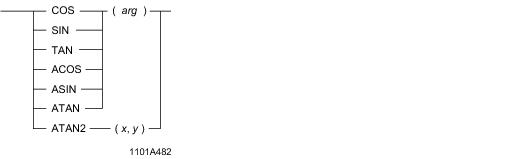# 15.00 - Trigonometric Functions (COS, SIN, TAN, ACOS, ASIN, ATAN, ATAN2) - Teradata Database

## Teradata Database SQL Functions, Operators, Expressions, and Predicates

Product
Release Number
15.00
Content Type
Programming Reference
Publication ID
B035-1145-015K
Language
English (United States)

## Trigonometric Functions (COS, SIN, TAN, ACOS, ASIN, ATAN, ATAN2)

### Purpose

Performs the trigonometric or inverse trigonometric function of an argument.

### Syntaxwhere:

 Syntax element … Specifies … arg any valid numeric expression that expresses an angle in radians. x the x-coordinate of a point to use in the arctangent calculation. y the y-coordinate of a point to use in the arctangent calculation.

### ANSI Compliance

This is a Teradata extension to the ANSI SQL:2011 standard.

### Definitions

 Function Definition Arccosine The arccosine is the angle whose cosine is the argument. Arcsine The arcsine is the angle whose sine is the argument. Arctangent The arctangent is the angle whose tangent is the argument. Cosine The cosine of an angle is the ratio of two sides of a right triangle. The ratio is the length of the side adjacent to the angle divided by the length of the hypotenuse. Sine The sine of an angle is the ratio of two sides of a right triangle. The ratio is the length of the side opposite to the angle divided by the length of the hypotenuse. Tangent The tangent of an angle is the ratio of two sides of a right triangle. The ratio is the length of the side opposite to the angle divided by the length of the side adjacent to the angle.

### Result Type and Attributes

Here are the default data type, format, and title for the result of the trigonometric and inverse trigonometric functions.

 Data Type Format Title FLOAT Default format for FLOAT Cos(arg)Sin(arg)Tan(arg)ArcCos(arg)ArcSin(arg)ArcTan(arg)Atan2(x,y)

For information on default data type formats, see SQL Data Types and Literals.

### Result Value

 Function Result Value COS(arg) The cosine of arg in radians in the range -1 to 1, inclusive. SIN(arg) The sine of arg in radians in the range -1 to 1, inclusive. TAN(arg) The tangent of arg in radians. ACOS(arg) An angle in the range 0 to π radians, inclusive. ASIN(arg) An angle in the range -π/2 to π/2 radians, inclusive. ATAN(arg) An angle in the range -π/2 to π/2 radians, inclusive. ATAN2(x,y) An angle between -π and π radians, excluding -π. A positive result represents a counterclockwise angle from the x-axis. A negative result represents a clockwise angle. ATAN2(x,y) equals ATAN(y/x), except that x can be 0 in ATAN2(x,y) and x cannot be 0 in ATAN(y/x) since this results in a divide by zero error. If both x and y are 0, an error is returned.

### Argument Types and Rules

Arguments that are not FLOAT are converted to FLOAT based on implicit type conversion rules. If an argument cannot be converted, an error is reported. For more information on implicit type conversion, see “Implicit Type Conversions” on page 583.

If an argument is a UDT, the following rules apply:

• The UDT must have an implicit cast to any of the following predefined types:
• Numeric
• Character
• DATE
• To define an implicit cast for a UDT, use the CREATE CAST statement and specify the AS ASSIGNMENT clause. For more information on CREATE CAST, see SQL Data Definition Language.

• Implicit type conversion of UDTs for system operators and functions, including trigonometric and inverse trigonometric functions, is a Teradata extension to the ANSI SQL standard. To disable this extension, set the DisableUDTImplCastForSysFuncOp field of the DBS Control Record to TRUE. For details, see Utilities: Volume 1 (A-K).
• Trigonometric and inverse trigonometric functions cannot take the following types of arguments:

• BYTE or VARBYTE
• BLOB or CLOB
• CHARACTER or VARCHAR if the server character set is GRAPHIC
• ### Examples

The following are representative function expressions and results.

 Expression Result COS(5-4) 5.40302305868140E -001 SIN(LOG(0.5)) -2.96504042171437E -001 SIN(RADIANS(180.0)) 1.22464679914735E-016 TAN(ABS(-3)) -1.42546543074278E -001 ACOS(-0.5) 2.09439510239320E 000 ASIN(1) 1.57079632679490E 000 ATAN(1+2) 1.24904577239825E 000 ATAN2(1,1) 7.85398163397448E -001